# Next Greater Element in an array. [NGE]

By | December 28, 2017

Question: There is an array A[N] of N numbers. You have to compose an array Output[N] such that each element in Output[i] will tell the next greater element to the right in the original array. If there is no greater number to the right, then the output array should contain -1 in that position.

Array 1: {4, 6, 5, 4, 3, 4, 9, 8, 1}

Output: {6, 9, 9, 9, 4, 9, -1, -1, -1}

Let us try to understand the problem statement. We are given an input array that has some random numbers. We need to produce another output array that contains the ‘Next Greater Element’ for each of the corresponding numbers of the original array. If there does not exist a greater element to the right, we simply replace it with ‘-1’.

In the sample input let us try to look at the elements.

 Element Next Greater Element Reason 4 6 The next element to 4 6 9 The next elements 5, 4, 3, 4 are smaller than 6. 9 is greater 5 9 The next elements 4, 3, 4 are smaller than 5. 9 is greater 4 9 The next elements 3, 4, are smaller/equal to 4. 9 is greater 3 4 The next element greater is 4 4 9 The next element greater is 9 9 -1 The next elements 8, 1 are smaller than 9. Hence -1 8 -1 The next element 1 is smaller than 8. Hence -1 1 -1 There is no element to the right, so no element is greater. Hence -1

We can easily deduce that the last element of the output array will always be ‘-1’ since there will be no element greater than it on the right.

Simple approach to the problem:

An easy solution to the problem would be by using 2 loops.

• Iterate over each element of the array
• For each element iterate over the remaining elements and find the next greater element.
• Populate the new array in the process.

This method would have a time complexity of O(n2) and would not be an effective solution.

Optimized approach to the problem [Using stacks]:

If we look at the ‘Reason’ column of the sample input, we can sense a pattern that could help us reach a solution to the problem. Before reading the solution try to think about a solution using stacks.

Algorithm:

1. Create a stack and push the first number in the stack.
3. If the top of the stack is greater/equal than the current number. Add the number to the stack.
4. If the top of the stack is less than the current number. Keep on popping elements from the stack until the top of the stack is greater/equal to the current number OR the stack gets empty. The current number is the NGE of all the elements popped from the stack.
5. If the stack is not empty at the end of all iterations, mark the NGE of remaining items in stack as -1.

Let us try to visualize the algorithm.
Input Array: – 4, 6, 5, 4, 3, 4, 9, 8, 1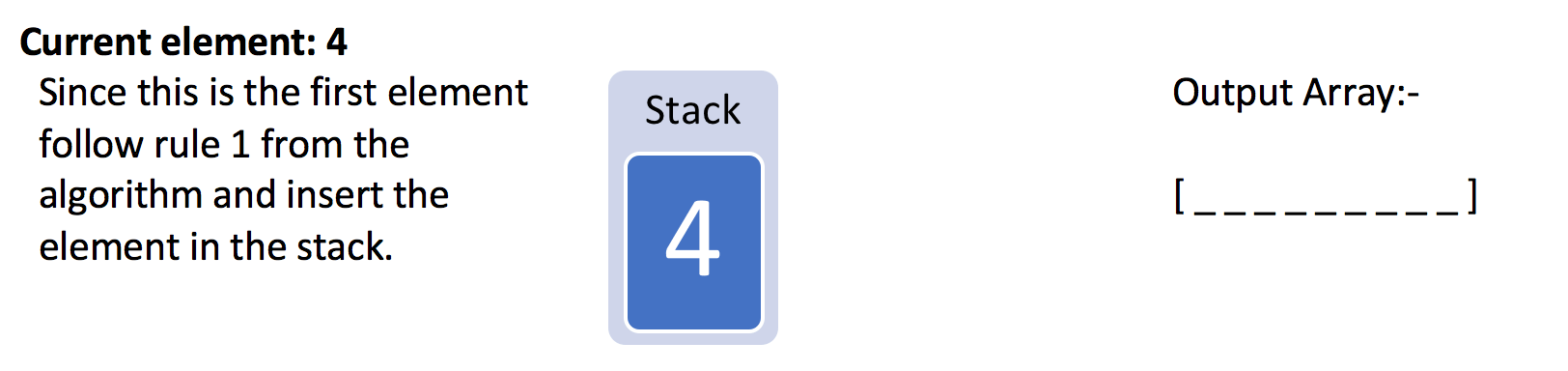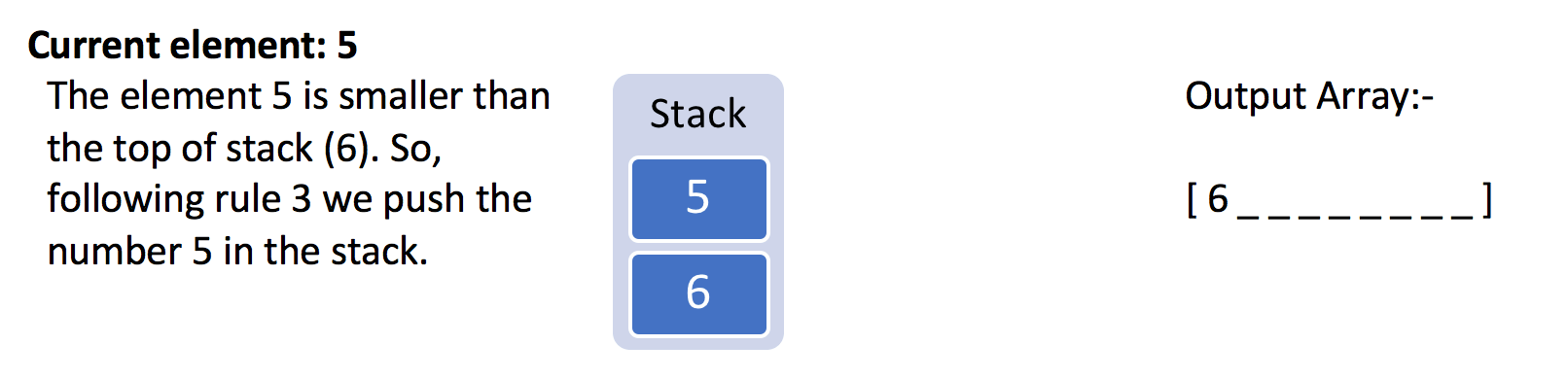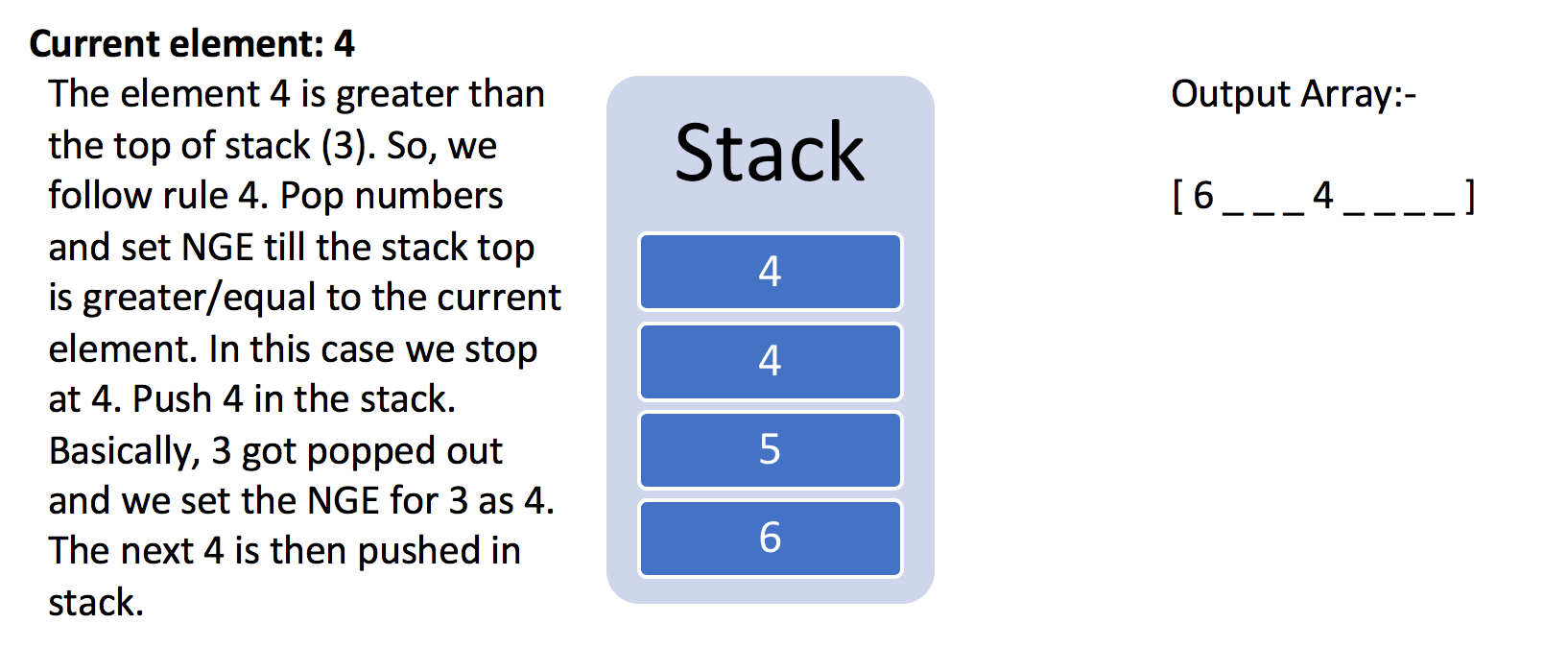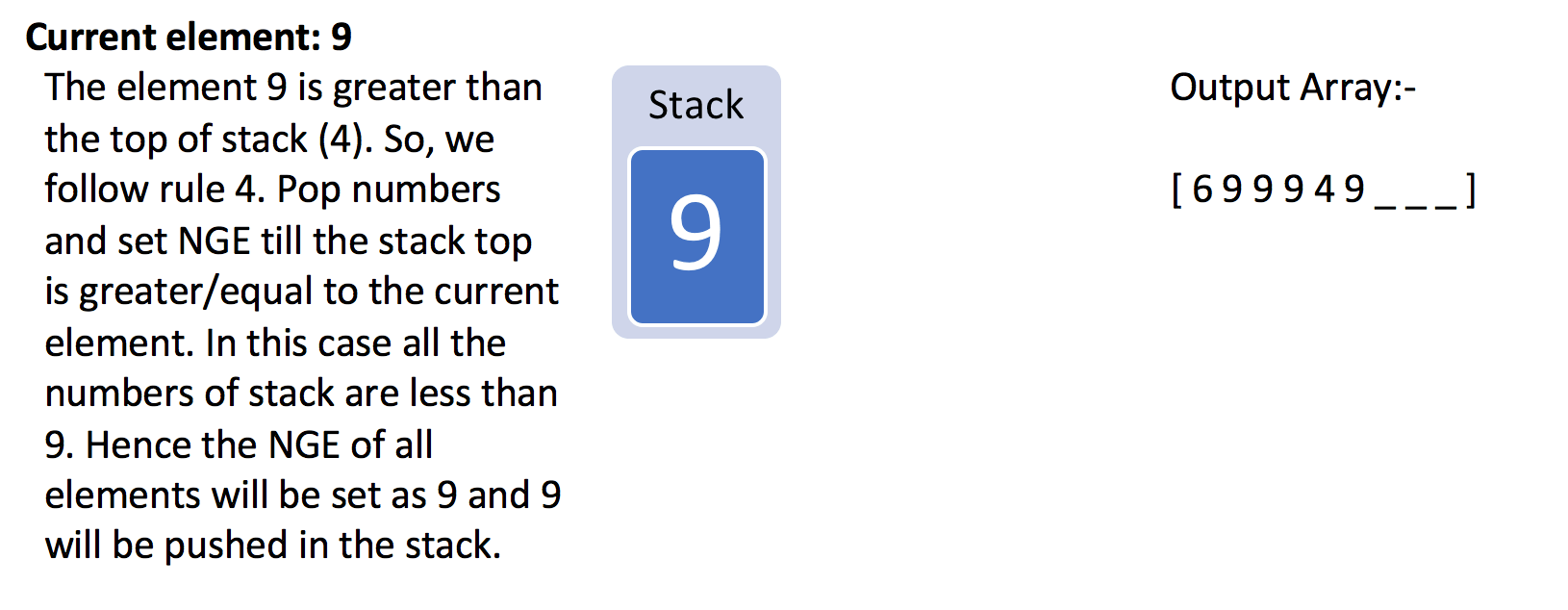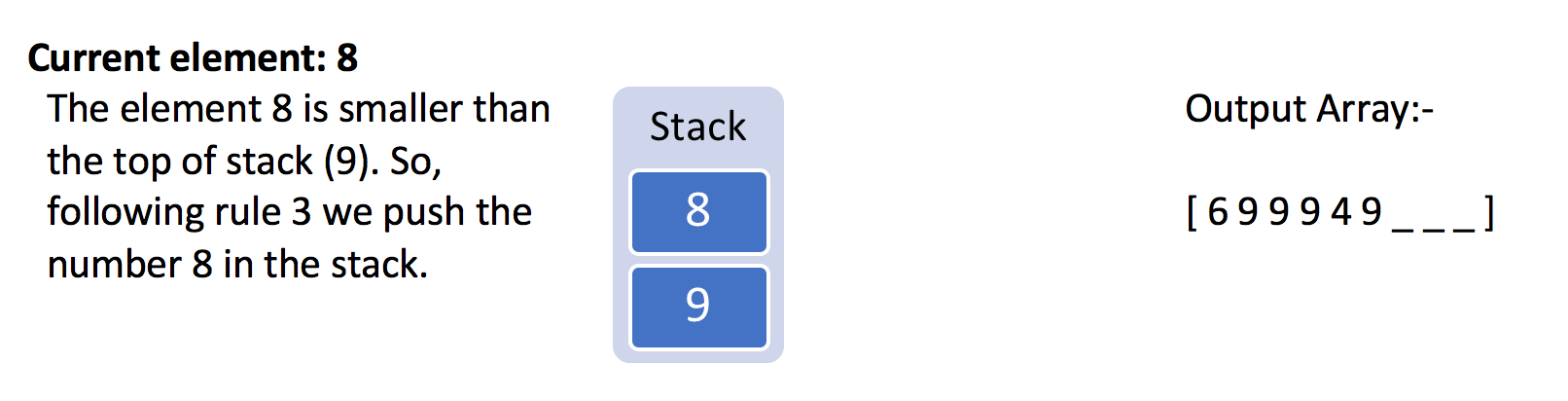Thus the output array contains the Next Greater Element for each of the element.
Below is an implementation based on a similar idea.

```public class NGE {

static int[] printNGE(int a[]) {

if (a == null) {
throw new NullPointerException();
}

if (a.length == 0) {
return new int;
}

if (a.length == 1) {
return new int[]{-1};
}

int[] indices = new int[a.length];
int[] nge = new int[a.length];

nge[a.length - 1] = -1;
indices[a.length - 1] = -1;

for (int i = a.length - 2; i >= 0; i--) {

int j = i + 1;
while (j != -1 && a[j] <= a[i]) {
j = indices[j];
}

indices[i] = j;
nge[i] = j == -1 ? -1 : a[j];
}

return nge;
}

public static void main(String[] args) {
int[] arr = {4, 6, 5, 4, 3, 4, 9, 8, 1};
for (int i : printNGE(arr)) {
System.out.print(i + " ");
}

}
```

A similar problem can be found for practice on Leetcode.Author: nikoo28

a tech-savvy guy and a design buff...

This site uses Akismet to reduce spam. Learn how your comment data is processed.